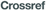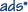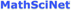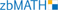0
2013
Impact Factor

# Features of Bifurcations of Periodic Solutions of the Ikeda Equation

2018, Vol. 14, no. 3, pp.  301-324

Author(s): Kubyshkin E. P., Moriakova A. R.

We study equilibrium states and bifurcations of periodic solutions from the equilibrium state of the Ikeda delay-differential equation well known in nonlinear optics. This equation was proposed as a mathematical model of a passive optical resonator in a nonlinear environment. The equation, written in a characteristic time scale, contains a small parameter at the derivative, which makes it singular. It is shown that the behavior of solutions of the equation with initial conditions from the fixed neighborhood of the equilibrium state in the phase space of the equation is described by a countable system of nonlinear ordinary differential equations. This system of equations has a minimal structure and is called the normal form of the equation in the neighborhood of the equilibrium state. The system of equations allows us to pick out one “fast” and a countable number of “slow” variables and apply the averaging method to the system obtained. It is shown that the equilibrium states of a system of averaged equations with “slow” variables correspond to periodic solutions of the same type of stability. The possibility of simultaneous bifurcation of a large number of stable periodic solutions(multistability bifurcation) is shown. With further increase in the bifurcation parameter each of the periodic solutions becomes a chaotic attractor through a series of period-doubling bifurcations. Thus, the behavior of the solutions of the Ikeda equation is characterized by chaotic multistability.
Keywords: Ikeda equation, periodic solutions, bifurcation of multistability, chaotic multistability
Citation: Kubyshkin E. P., Moriakova A. R., Features of Bifurcations of Periodic Solutions of the Ikeda Equation, Rus. J. Nonlin. Dyn., 2018, Vol. 14, no. 3, pp.  301-324
DOI:10.20537/nd180302

## References

 Ikeda, K., “Multiple-Valued Stationary State and Its Instability of the Transmitted Light a Ring Cavity System”, Opt. Commun., 30:2 (1979), 257–261Ikeda, K., Daido, H., and Akimoto, O., “Optical Turbulence: Chaotic Behavior of Transmitted Light from a Ring Cavity”, Phys. Rev. Lett., 45:9 (1980), 709–712Ikeda, K. and Matsumoto, K., “High-Dimensional Chaotic Behavior in System with Time-Delayed Feedback”, Phys. D, 29:1–2 (1987), 223–235Sprott, J. C., Elegant Chaos: Algebraically Simple Chaotic Flows, World Sci., Singapore, 2010, 304 pp.Ponomarenko, V. I. and Prokhorov, M. D., “Recovering Parameters of the Ikeda Equation from Noisy Time Series”, Tech. Phys. Lett., 31:3 (2005), 252–254; Pis'ma Zh. Tekh. Fiz., 31:6 (2005), 73–78 (Russian)Larger, L., Goedgebuer, J.-P., and Udaltsov, V., “Ikeda-Based Nonlinear Delayed Dynamics for Application to Secure Optical Transmission Systems Using Chaos”, C. R. Math. Acad. Sci. Paris, 5:6 (2004), 669–681
 Kubyshkin, E. P. and Nazarov, A. Yu., “Analysis of Oscillatory Solutions of a Nonlinear Singularly Perturbed Differential-Difference Equation”, Vestn. Nizhegorodsk. Univ., 5:2 (2010), 118–125 (Russian)
 Kubyshkin, E. P. and Moriakova, A. R., “Analysis of Bifurcations of Periodic Solutions of Ikeda Equation”, Nonlinear Phenomena in Complex Systems, 20:1 (2017), 40–49Bellman, R. and Cooke, K. L., Differential-Difference Equations, Acad. Press, New York, 1963, 462 pp.Krein, S. G, Linear Differential Equations in Banach Space, AMS, Providence, R.I., 1972Shimanov, S. N., “On the Vibration Theory of Quasilinear Systems with Lag”, J. Appl. Math. Mech., 23:5 (1959), 1198–1208; Prikl. Mat. Mekh., 23:5 (1959), 836–844 (Russian)Krasnosel'skii, M. A., Vainikko, G. M., Zabreyko, P. P., Ruticki, Ya. B., and Stetsenko, V. Ya., Approximate Solution of Operator Equation, Wolters-Noordhoff, Groningen, 1972, 496 pp.Neimark, Yu. I., “D-Partitions of the Space of Quasipolynomials (to the Stability of Linearized Distributed Systems)”, Prikl. Mat. Mekh., 13:4 (1949), 349–380 (Russian)Glyzin, D. S., The Program Package for the Analysis of Dynamic Systems “Tracer”, Appl. no. 2008610548 dated Feb 14, 2008, Certificate of State Registration of the Computer Program no. 2008611464, Registered in the Register of Computer Programs 24.03.2008 (Russian)